## Low-Light Image Enhancement

Images captured in outdoor scenes can be highly degraded due to poor lighting conditions. These images can have low dynamic ranges with high noise levels that affect the overall performance of computer vision algorithms. To make computer vision algorithms robust in low-light conditions, use low-light image enhancement to improve the visibility of an image. The histogram of pixel-wise inversion of low-light images or HDR images is very similar to the histogram of hazy images. Thus, you can use haze removal techniques to enhance low-light images.

Using haze removal techniques to enhance low-light images comprises three steps:

• Step 1: Invert the low-light image.

• Step 2: Apply the haze removal algorithm to the inverted low-light image.

• Step 3: Invert the enhanced image.

### Enhance Low Light Image using Dehazing Algorithm

Import an RGB image captured in low light.

```A = imread('lowlight_11.jpg'); figure, imshow(A);```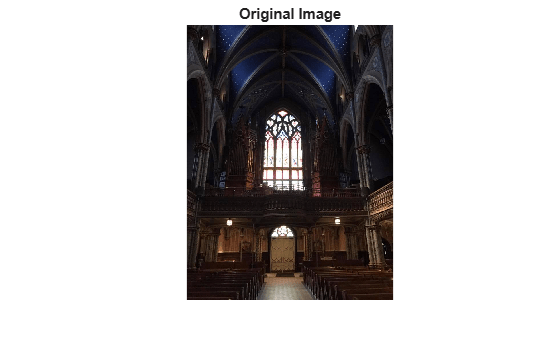Invert the image and notice how the low-light areas in the original image appear hazy.

```AInv = imcomplement(A); figure, imshow(AInv);```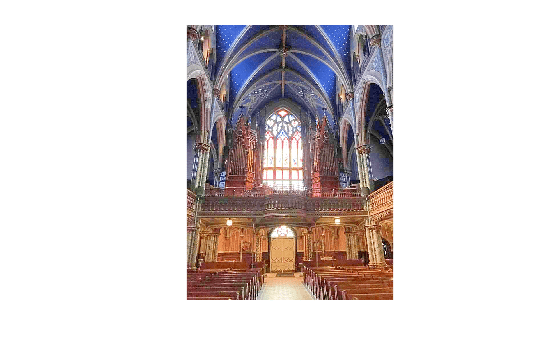Reduce the haze using the `imreducehaze` function.

```BInv = imreducehaze(AInv); figure, imshow(BInv);```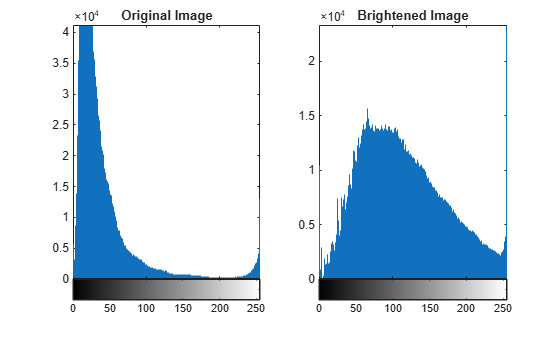Invert the results to obtain the enhanced image.

`B = imcomplement(BInv);`

Display the original image and the enhanced images, side-by-side.

`figure, montage({A, B});`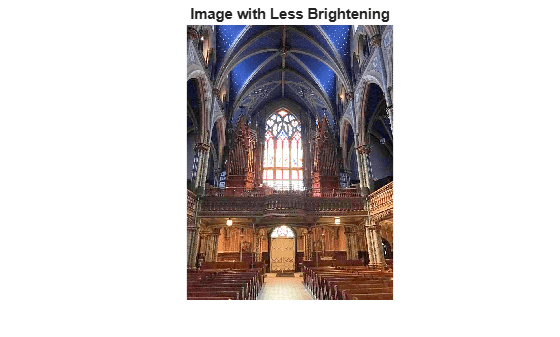### Improve Results Further Using `imreducehaze` Optional Parameters

To get a better result, call `imreducehaze` on the inverted image again, this time specifying some optional parameters.

```BInv = imreducehaze(AInv, 'Method','approx','ContrastEnhancement','boost'); BImp = imcomplement(BInv); figure, montage({A, BImp});```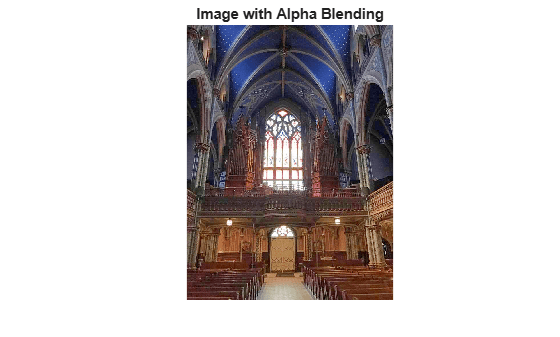### Another Example of Improving a Poorly Lit Image

Import an RGB image captured in low light.

`A = imread('lowlight_21.jpg');`

Invert the image.

`AInv = imcomplement(A);`

Apply the dehazing algorithm.

`BInv = imreducehaze(AInv, 'ContrastEnhancement', 'none');`

Invert the results.

`B = imcomplement(BInv);`

Display the original image and the enhanced images, side-by-side.

`figure, montage({A, B});`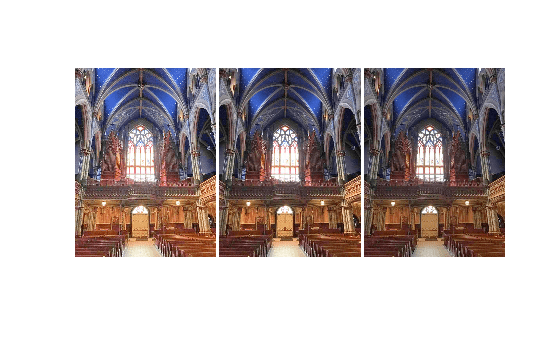### Reduce Color Distortion by using a Different Color Space

Convert the input image from the RGB colorspace to the L*a*b* colorspace.

`Lab = rgb2lab(A);`

Invert the L*a*b* image.

`LInv = imcomplement(Lab(:,:,1) ./ 100);`

Dehaze the inverted image using the `imreducehaze` function.

`LEnh = imcomplement(imreducehaze(LInv,'ContrastEnhancement','none'));`

Increase the saturation.

```LabEnh(:,:,1) = LEnh .* 100; LabEnh(:,:,2:3) = Lab(:,:,2:3) * 2; % Increase saturation```

Convert the image back to an RGB image and display the original and the enhanced image, side-by-side.

```AEnh = lab2rgb(LabEnh); figure, montage({A, AEnh});```### Improve Results using Denoising

Low-light images can have high noise levels. Enhancing low-light images can increase this noise level. Denoising can be a useful post-processing step.

Use the `imguidedfilter` function to remove noise from the enhanced image.

```B = imguidedfilter(BImp); figure, montage({BImp, B});```### Estimate Illumination Map

Import an RGB image captured in low light.

`A = imread('lowlight_21.jpg');`

Invert the image.

`AInv = imcomplement(A);`

Apply the dehazing algorithm to the image.

`[BInv, TInv] = imreducehaze(AInv, 'Method', 'approxdcp', 'ContrastEnhancement', 'none');`

Invert the enhanced image.

`T = imcomplement(TInv);`

Display the original image next to the estimated illumination map in false color.

```figure, subplot(1,2,1); imshow(A), title('Lowlight Image'); subplot(1,2,2); imshow(T), title('Illumination Map'); colormap(gca, hot(256));```### Limitations

This method can lose some details or get over-enhanced because of poor adaptability of the dark channel in low-light conditions.

### References

Dong, Xuan, et al. "Fast efficient algorithm for enhancement of low lighting video." Multimedia and Expo (ICME), 2011 IEEE International Conference on. IEEE, 2011.

 Dong, X., G. Wang, Y. Pang, W. Li, J. Wen, W. Meng, and Y. Lu. "Fast efficient algorithm for enhancement of low lighting video." Proceedings of IEEE® International Conference on Multimedia and Expo (ICME). 2011, pp. 1–6.

﻿